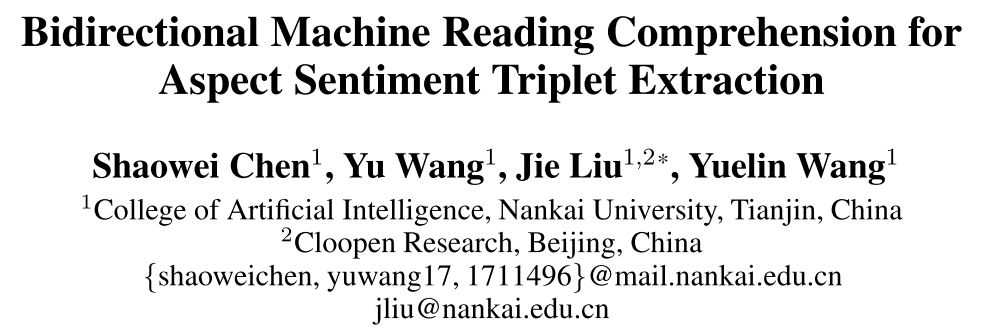# 1. ASTE任务

Aspect Sentiment Triplet Extraction（ASTE）是细粒度观点挖掘任务，它包含多个子任务，比如观点实体抽取、关系检测、情感分类等。作者此次的目的是从Review中抽取出aspect-opinion-sentiment triplet。

ASTE任务是由Peng等人在2020AAAI——Knowing What, How and Why: A Near Complete Solution for Aspect-Based Sentiment Analysis中提出的。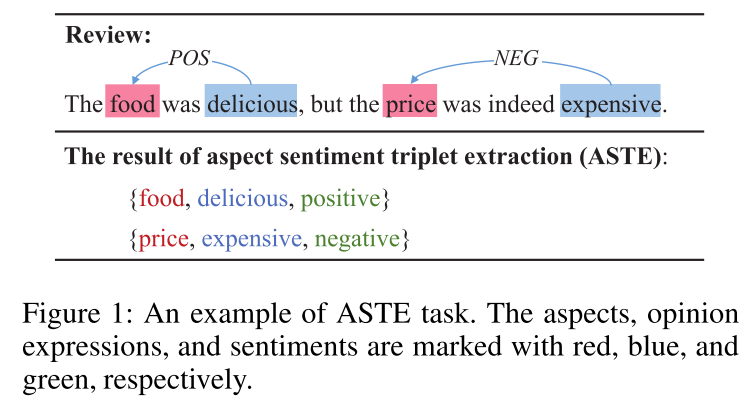# 3. 论文贡献

• 作者把ASTE任务作为三轮机器阅读理解任务来做，在一个统一的框架下优雅的标识出句中的triplet；
• 提出了双向MRC框架；
• 进行了大量实验并达到了SOTA效果。

# 4. 方法介绍

## 4.1 整体框架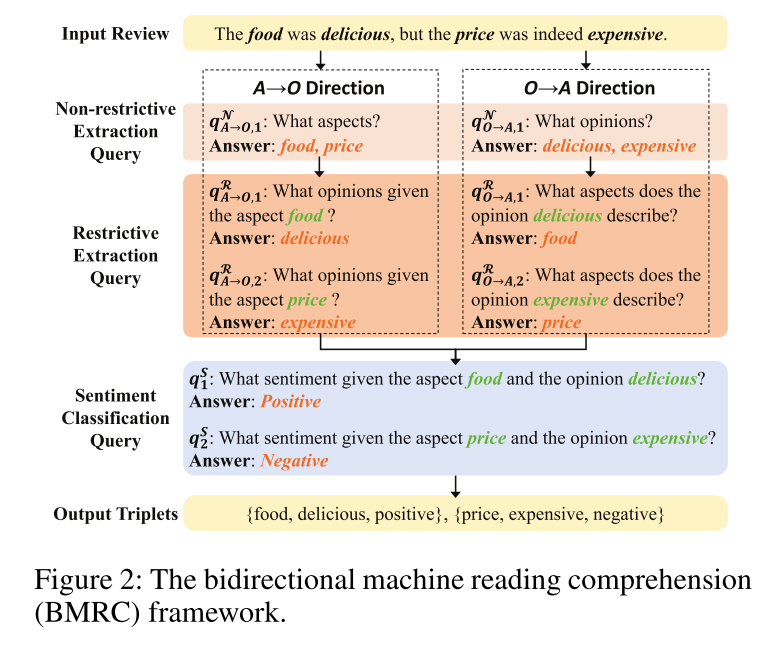## 4.2 问题定义

• 非限制性抽取queries $Q^{\mathcal N} = \{q_i^{\mathcal N}\}_{i=1}^{|Q^{\mathcal N}|}$
• 限制性抽取queries $Q^{\mathcal R} = \{q_i^{\mathcal R}\}_{i=1}^{|Q^{\mathcal R}|}$
• 情感分类queries $Q^{\mathcal S} = \{q_i^{\mathcal S}\}_{i=1}^{|Q^{\mathcal S}|}$

## 4.3 Query构造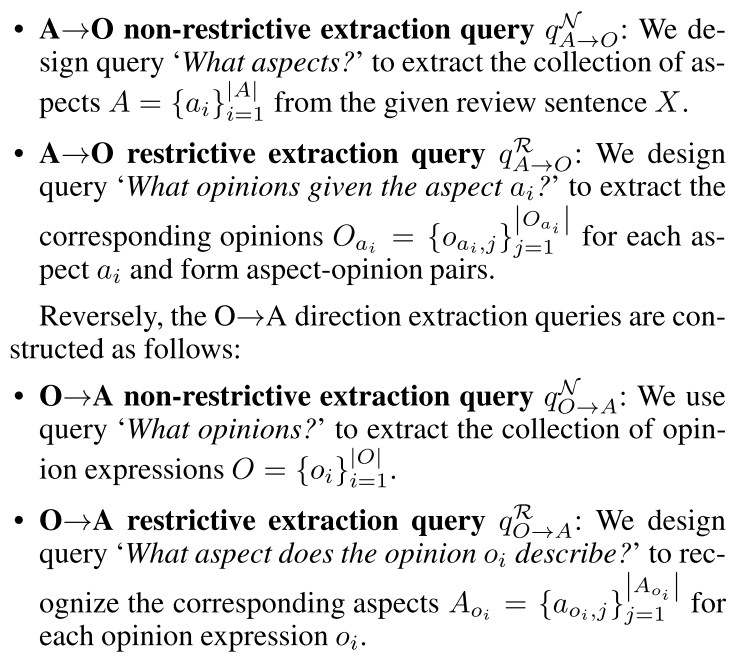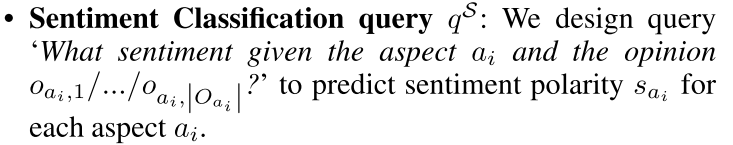## 4.4 编码层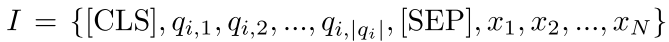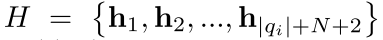## 4.5 答案预测

Answer for Extraction Query.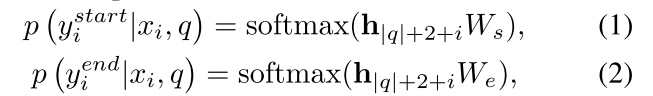Answer for Sentiment Classification Query.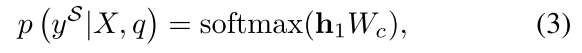## 4.6 联合学习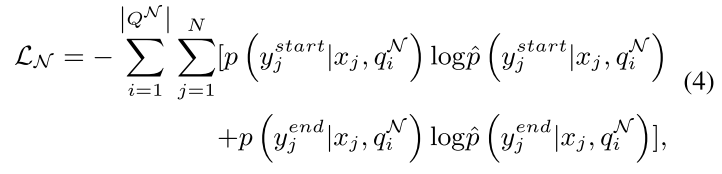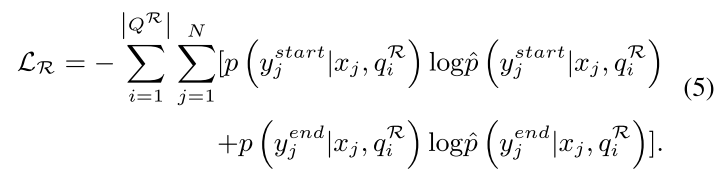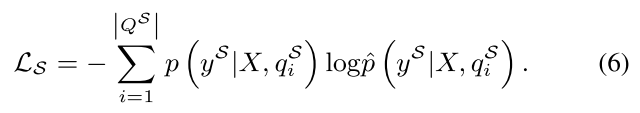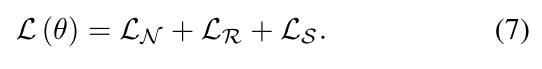## 4.7 推理

$A \rightarrow O$方向，由非限制性抽取query $q_{A \rightarrow O}^{\mathcal N}$标识出aspect 集合$A=\{a_1, a_2, ..., a_{|A|}\}$。对于每一个aspect $a_i$，限制性抽取query $q_{A \rightarrow O}^{\mathcal R}$标识出相对应的opinion集合（一个aspect可能对应多个opinion）。由此，得到aspect-opinion pairs $V_{A\rightarrow O}=[(a_k, o_k)]_{k=1}^K$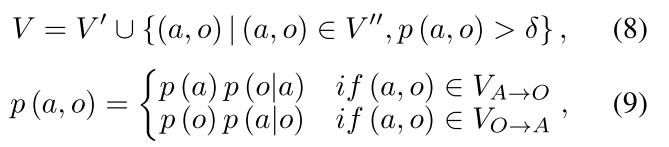$V'$表示两个方向的$V$的交集，$V''$表示差集。对于差集中的pair，只有概率大于阈值$\delta$才算正确。